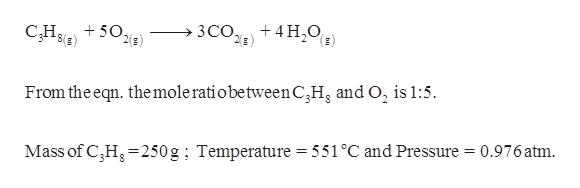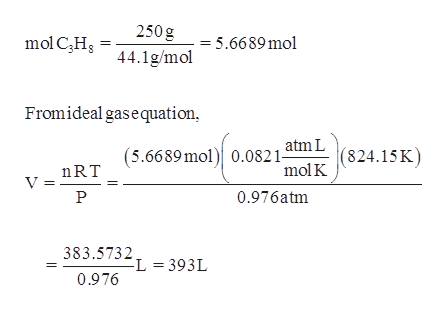# Given the following reaction, what volume of oxygen would be needed to react with 250. grams of propane at 551 °C and 0.976 atm?C3H8 (g) + 5 O2 (g) -> 3 CO2 (g) + 4 H2O (g)

Question
1 views

Given the following reaction, what volume of oxygen would be needed to react with 250. grams of propane at 551 °C and 0.976 atm?
C3H8 (g) + 5 O2 (g) -> 3 CO2 (g) + 4 H2O (g)

check_circle

Step 1

Given information,help_outlineImage TranscriptioncloseЗСО) 4 H O C,H 502 and O2 is 1:5. From the eqn. themole ratiobetweenC3H Mass of CH250g; Temperature = 551°C and Pressure = 0.976 atm fullscreen
Step 2

Calculate the volume...help_outlineImage Transcriptionclose250 g mol CHs = 5.6689 mol 44.1g/mol Fromideal gasequation atm L (824.15K mol K (5.6689mol 0.082 1- nRT V = 0.976atm 383.5732 L 393L 0.976 fullscreen

### Want to see the full answer?

See Solution

#### Want to see this answer and more?

Solutions are written by subject experts who are available 24/7. Questions are typically answered within 1 hour.*

See Solution
*Response times may vary by subject and question.
Tagged in

### Chemistry